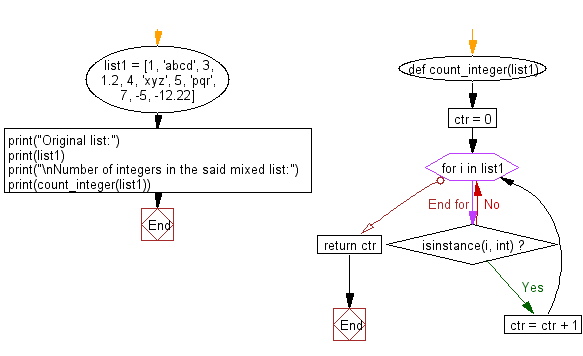﻿ Python: Count integer in a given mixed list - w3resource# Python: Count integer in a given mixed list

## Python List: Exercise - 106 with Solution

Write a Python program to count integer in a given mixed list.

Sample Solution:

Python Code:

``````def count_integer(list1):
ctr = 0
for i in list1:
if isinstance(i, int):
ctr = ctr + 1
return ctr

list1 = [1, 'abcd', 3, 1.2, 4, 'xyz', 5, 'pqr', 7, -5, -12.22]

print("Original list:")
print(list1)

print("\nNumber of integers in the said mixed list:")
print(count_integer(list1))
```
```

Sample Output:

```Original list:
[1, 'abcd', 3, 1.2, 4, 'xyz', 5, 'pqr', 7, -5, -12.22]

Number of integers in the said mixed list:
6
```

Flowchart:## Visualize Python code execution:

The following tool visualize what the computer is doing step-by-step as it executes the said program:

Python Code Editor:

Have another way to solve this solution? Contribute your code (and comments) through Disqus.

What is the difficulty level of this exercise?

Test your Python skills with w3resource's quiz

﻿

## Python: Tips of the Day

```print(2_000_000)
```2000000